# Cancel fractions

Compress the expression of factorial:

(n+6)!/(n+4)!-n!/(n-2)!

Result

e = (Correct answer is: 12n+30)#### Solution:

$e=(n+6)! / (n+4)! -n!/ (n-2)! \ \\ e=(n+6)(n+5) (n+4)! / (n+4)! -n(n-1) (n-2)! / (n-2)! \ \\ e=(n+6)(n+5)-n(n-1) \ \\ e=n^2 +6n+5n+6 \cdot \ 5 -n^2-n \ \\ e=12n+30$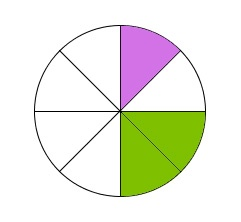Our examples were largely sent or created by pupils and students themselves. Therefore, we would be pleased if you could send us any errors you found, spelling mistakes, or rephasing the example. Thank you!

Leave us a comment of this math problem and its solution (i.e. if it is still somewhat unclear...):Be the first to comment!Tips to related online calculators
Need help calculate sum, simplify or multiply fractions? Try our fraction calculator.
Would you like to compute count of combinations?

## Next similar math problems:How many ways can we thread 4 red, 5 blue, and 6 yellow beads onto a thread?
2. Three workplacesHow many ways can we divide nine workers into three workplaces if they need four workers in the first workplace, 3 in the second workplace and 2 in the third?
3. Math logicThere are 20 children in the group, each two children have a different name. Alena and John are among them. How many ways can we choose 8 children to be among the selected A) was John B) was John and Alena C) at least one was Alena, John D) maximum one
4. PrizeHow many ways can be rewarded 9 participants with the first, second and third prize in a sports competition?
5. Five letters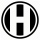How many ways can five letters be arranged?
6. Possible combinations - word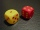How many ways can the letters F, A, I, R be arranged?
7. Bookshelf and books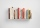How many ways can we place 7 books in a bookshelf?
8. Practice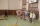How many ways can you place 20 pupils in a row when starting on practice?
9. CombinationsIf the number of elements increase by 3, it increases the number of combinations of the second class of these elements 5 times. How many are the elements?
10. Value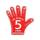Find the value of the expression: 6!·10^-3
11. ClassroomOf the 26 pupils in the classroom, 12 boys and 14 girls, four representatives are picked to the odds of being: a) all the girls b) three girls and one boy c) there will be at least two boys
12. Boys and girlsThere are eight boys and nine girls in the class. There were six children on the trip from this class. What is the probability that left a) only boys b) just two boys
13. Combinations 66 purses 9 flaps 12 straps Every combination must include 1 purse, 1 flap, and 1 strap. How many are possible combinations?
14. VCP equationSolve the following equation with variations, combinations and permutations: 4 V(2,x)-3 C(2,x+ 1) - x P(2) = 0
15. LunchSeven classmates go every day for lunch. If they always come to the front in a different order, will be enough school year to take of all the possibilities?
16. Desks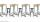A class has 20 students. The classroom consists of 20 desks, with 4 desks in each of 5 different rows. Amy, Bob, Chloe, and David are all friends, and would like to sit in the same row. How many possible seating arrangements are there such that Amy, Bob,
17. Elements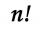If the number of elements is decreased by two the number of permutations is decreased 30 times. How many elements are?